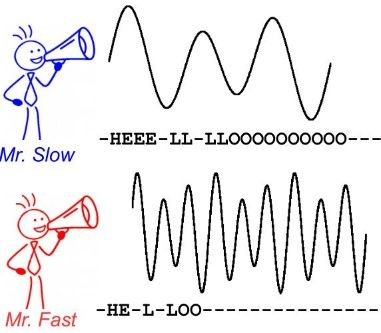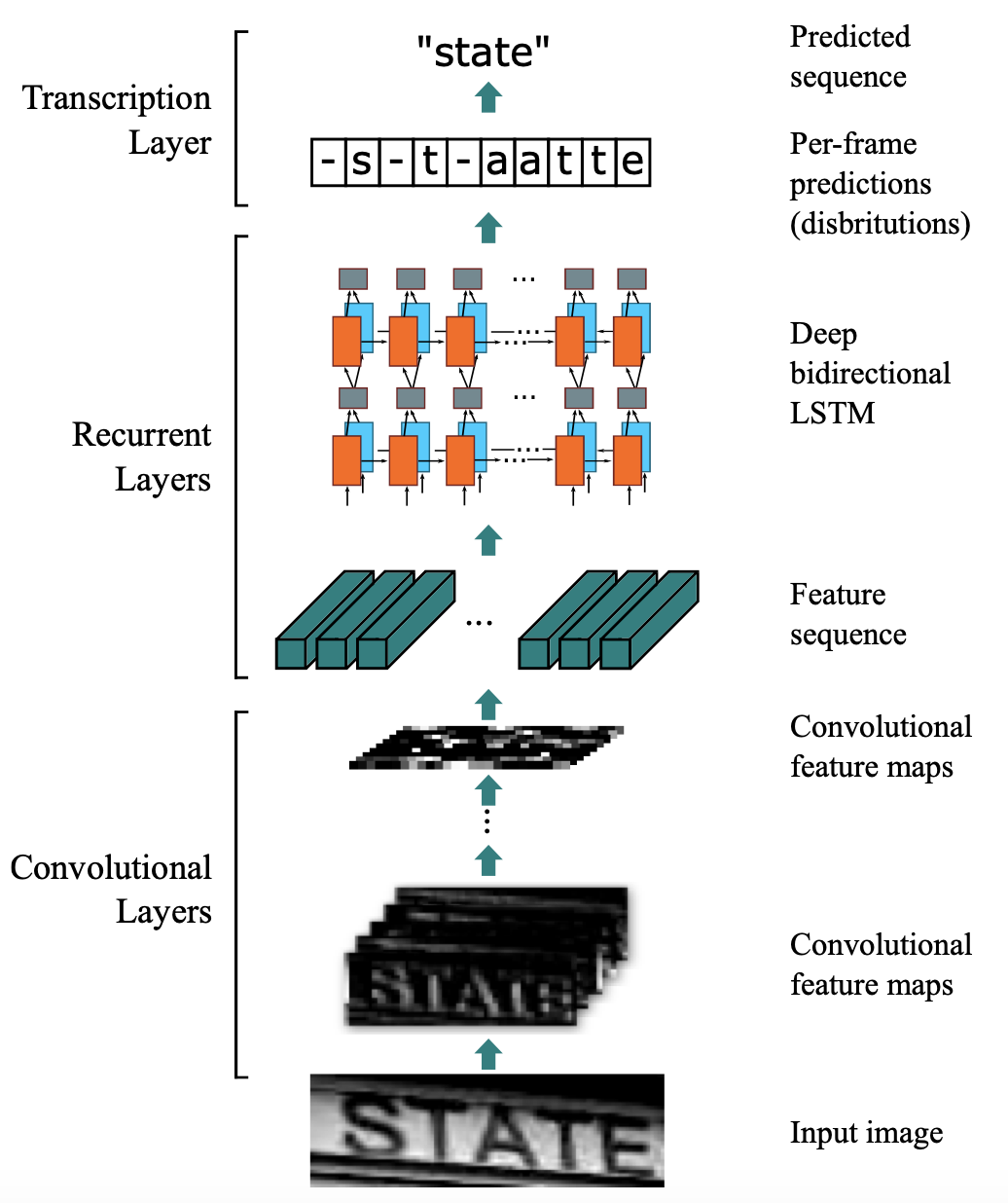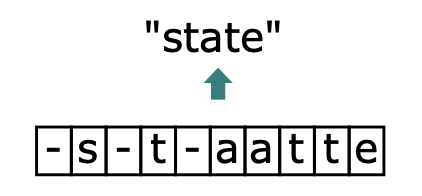# CTC算法¶

## 算法背景¶

CTC 算法主要用来解决神经网络中标签和预测值无法对齐的情况，通常用于文字识别以及语音等序列学习领域。举例来说，在语音识别任务中，我们希望语音片段可以与对应的文本内容一一对应，这样才能方便我们后续的模型训练。但是对齐音频与文本是一件很困难的事，如 图1 所示，每个人的语速都不同，有人说话快，有人说话慢，我们很难按照时序信息将语音序列切分成一个个的字符片段。而手动对齐音频与字符又是一件非常耗时耗力的任务。1. $$X$$$$Y$$ 都是变长的；

2. $$X$$$$Y$$ 的长度比也是变化的；

3. $$X$$$$Y$$ 相应的元素之间无法严格对齐。

## 算法概述¶CRNN中，首先使用CNN提取图片特征，特征图的维度为$$m\times T$$ ，特征图 $$x$$ 可以定义为：

$x = (x^1,x^2,...,x^T)$

$x^t = (x_1^t,x_2^t,...,x_m^t)$

$y = (y^1,y^2,...,y^T)$

$y^t = (y_1^t,y_2^t,...,y_n^t)$

$$n$$ 为字符字典的长度，由于 $$y_i^t$$ 是概率，所以 $$\Sigma_i{y_i^t}=1$$ 。对每一列 $$y^t$$$$argmax()$$ ，就可以获取每个类别的概率。

$L' = L \cup \{-\}$$B(-s-t-aatte)=state$
$B(ss-t-a-t-e)=state$
$B(sstt-aat-e)=state$

$B(-s-t-tatte)=sttate$

$p(l|x) = \Sigma_{\pi\in B^{-1}(l)}p(\pi|x)$

$p(\pi|x) = \Pi_{t=1}^Ty^t_{\pi_t}$

$\pi = -s-t-aatte$
$y^t_{\pi_t} = y_-^1*y_s^2*y_-^3*y_t^4*y_-^5*y_a^6*y_a^7*y_t^8*y_t^9*y_e^10$